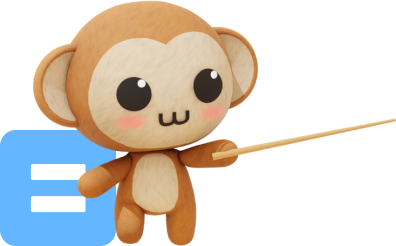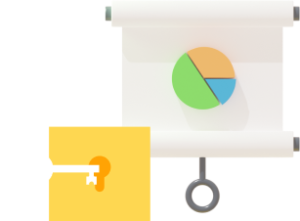# Primary 2 Syllabus

Aligned with the latest 2021 Singapore MOE Math syllabus and endorsed by Education Alliance of Finland.
Hundreds of hours of learning content for all topics covered in P2 to get your child build a strong Math foundation!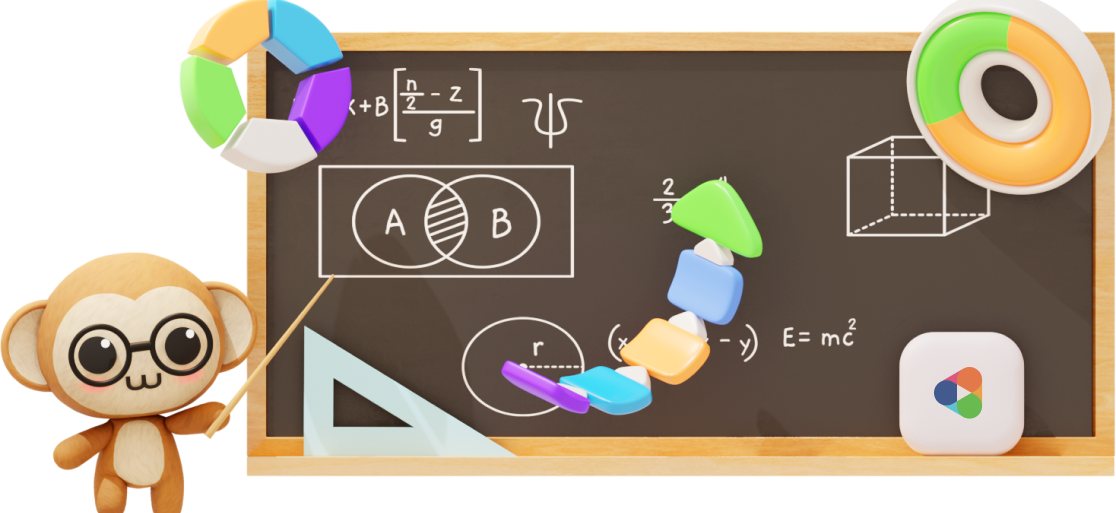## Overview

To help your child form a positive attitude towards learning math, we make it a fun and progressive experience:

• Collect virtual pets as they practice and complete quests to upgrade their accessories.
• Challenge friends to math duels, or team up to solve problems together.
• Learn at their own pace!

They won’t get boring lessons in our system.

They won’t get cookie-cutter “assessment book style” questions.

They WILL get lots of enjoyment as we introduce them to the world of mathematics and build their foundations for future exploration!

Our syllabus is 100% aligned with MOE, and the Education Alliance of Finland (EAF) has also certified us for educational quality and effectiveness.

### Features##### Gamification to make learning engaging and rewarding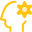##### A.I. + Practicle system to personalise questions to your child’s ability### Primary 2 Math Syllabus – What’s in it

Practicle’s online Math questions cover all topics and skills in the Singapore Primary 2 Math curriculum. Here’s a list of all the P2 topics and skills that are tested in our multiple-choice questions (MCQs) and word problem sums.

The 2021 Primary 2 Math Syllabus can be divided into 3 main branches – Numbers, Measurement and Geometry and finally, Graphs. In each branch, the Math questions will be divided into 3 difficulty levels – easy, medium and hard.

#### P2 Math: Numbers

Math for Primary 2 kids builds on what your child has learnt in Primary 1 and expands the range of numbers that they deal with.

The Primary 2 (P2) Math topics that are covered under Number are Numbers, Addition, Subtraction, Multiplication, Division and Fractions. You can refer below for the breakdown of the skills in each topic.#### Whole Numbers

• Count the number of objects in hundreds (Max:1000)
• Compare and order numbers (Max:1000)
• Understand place values up to 100• Add a 1-digit number to 3-digit number
• Add a 2-digit number to 3-digit number#### Subtraction

• Subtract a 1-digit number to 3-digit number
• Subtract a 2-digit number to 3-digit number
• Subtract 2 3-digit numbers#### Multiplication

• 2 Multiplication table
• 3 Multiplication table
• 4 Multiplication table
• 5 Multiplication table
• 10 Multiplication table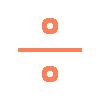#### Division

• Write an equation with a division sign
• Divide by 2
• Divide by 3
• Divide by 4
• Divide by 5
• Divide by 10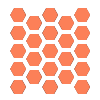#### Patterns

• Identify odd & even number sequences
• Identify patterns with 2D shapes according to size, shape, colour & orientation
• Identify patterns with 3D shapes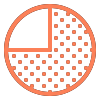#### Fractions

• Write fractions
• Compare and order fractions (unit fractions)
• Compare and order fractions (like fractions)
• Add fractions within a whole (like fractions)
• Subtract fractions within a whole (like fractions)#### Money

• Read and write money in decimal notation
• Compare money
• Express money in decimal to cents only and vice versa

#### P2 Math: Measurement and Geometry

The Primary 2 (P2) Math topics that are covered under Measurement and Geometry are expansions on what the Primary 1s have learnt about Shapes, Length, Time and Money. New topics include Solids, Mass and Volume. See below for the breakdown of the skills in each topic.#### Length

• Estimate length of more than 1 m or less
• Choose when to use cm vs m
• Reading length with a ruler
• Compare and order lengths#### Time

• Tell time to 5 minutes
• Use ‘a.m.’ and ‘p.m.’
• Use h and min
• Identify hands on the clock face for a given time (mins)
• Identify hands on the clock face for a given time (hrs)
• Measure duration of 1 hour/half hour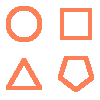#### 2D Shapes

• Identify a semi-circle
• Identify a quarter circle#### Mass

• Estimate mass of more than 1 kg or less
• Choose when to use g vs kg
• Reading mass with a scale
• Compare and order mass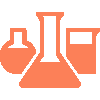#### Volume

• Estimate volume of more than 1 ℓ or less
• Compare and order volume#### 3D Shapes

• Identify a cube
• Identify a cuboid
• Identify a cylinder
• Identify a cone
• Identify a sphere

#### P2 Math: Graphs

The Primary 2 (P2) Math topics that are covered under Measurement and Geometry are expansions on what the Primary 1s have learnt about Shapes, Length, Time and Money. New topics include Solids, Mass and Volume. See below for the breakdown of the skills in each topic.#### Data Analysis

• Read and interpret picture graphs with scales

### Sample P2 questions and video explanations### Experience Practicle free, no commitment no hidden cost no lock-in

Try our learning platform free with a 7 day trial
and see if your child likes it.